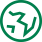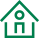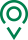• EN
• 圣康首页
• {if condition="\$categorys[\$vo]['is_menu'] == 1"}
• 关于圣康
• {if condition="\$categorys[\$vo]['is_menu'] == 1"} {if condition="\$categorys[\$vo]['is_menu'] == 1"}
• 产品展示
• {if condition="\$categorys[\$vo]['is_menu'] == 1"} {if condition="\$categorys[\$vo]['is_menu'] == 1"}
• 荣誉资质
• {if condition="\$categorys[\$vo]['is_menu'] == 1"} {if condition="\$categorys[\$vo]['is_menu'] == 1"}
• 新闻资讯
• {if condition="\$categorys[\$vo]['is_menu'] == 1"} {if condition="\$categorys[\$vo]['is_menu'] == 1"}
• 联系我们
• {if condition="\$categorys[\$vo]['is_menu'] == 1"} {if condition="\$categorys[\$vo]['is_menu'] == 1"}
• 在线留言
• {if condition="\$categorys[\$vo]['is_menu'] == 1"} {if condition="\$categorys[\$vo]['is_menu'] == 1"}
• 质量管控
• {if condition="\$categorys[\$vo]['is_menu'] == 1"} {if condition="\$categorys[\$vo]['is_menu'] == 1"}
• 源头种植
• {if condition="\$categorys[\$vo]['is_menu'] == 1"} {if condition="\$categorys[\$vo]['is_menu'] == 1"}
• 过程控制
• {if condition="\$categorys[\$vo]['is_menu'] == 1"}

天冷了，果蔬牛羊肉涨价

从环比看，10月份本市CPI环比由涨转降，主要受食品价格季节性下降所致，其中虾蟹类、薯类、鲜菜、鸡蛋和淡水鱼价格分别下降11.4%、9.9%、8.9%、6.9%和5.0%，共计影响CPI下降约0.4个百分点。受货源减少、需求增加影响，羊肉价格环比上涨3.1%；受猪瘟疫情影响，猪肉价格下旬有所回落。在外餐饮价格上涨0.1%，今年以来几乎均处于上涨区间。

统计还显示，本市其他七大类价格环比“六涨一降”。其中，衣着、交通和通信、其他用品和服务价格分别上涨0.9%、0.8%和0.5%；生活用品及服务、医疗保健价格分别上涨0.2%、0.1%；教育文化和娱乐价格持平；居住价格下降0.1%。

我们的宗旨

•全球精选

一站式购物方案

•多仓直发

特有次日达配送服务

•源头直采

精选正宗好食材

•天天底价

新鲜到家购不停